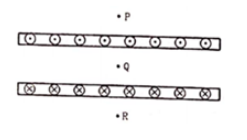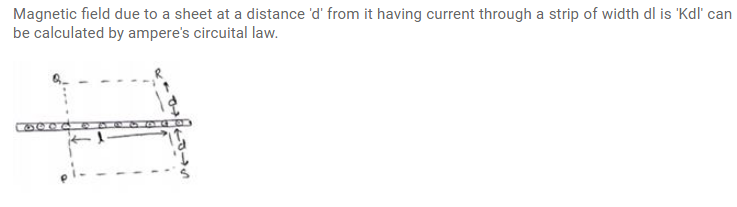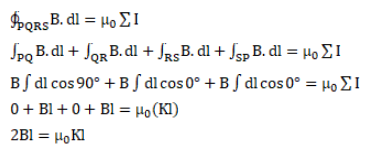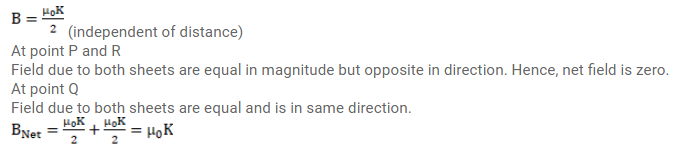# Two large metal sheets carry surface currents as shown in figure.

Question:

Two large metal sheets carry surface currents as shown in figure. The current through a strip of width dl is $\mathrm{Kdl}$ where $\mathrm{K}$ is constant. Find the magnetic field at the points $\mathrm{P}, \mathrm{Q}$ and $\mathrm{R}$.Solution: